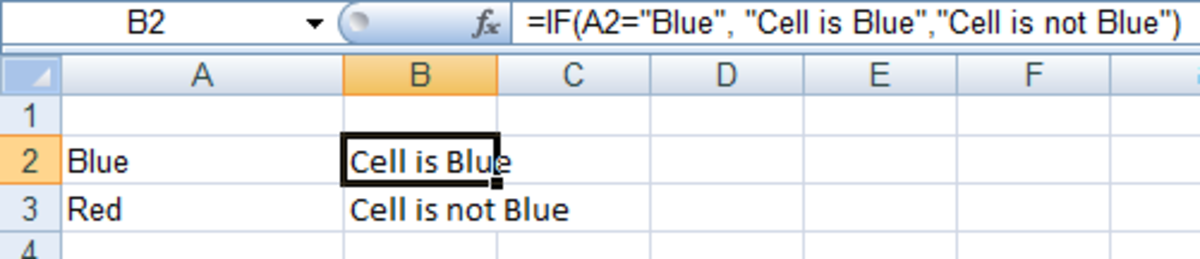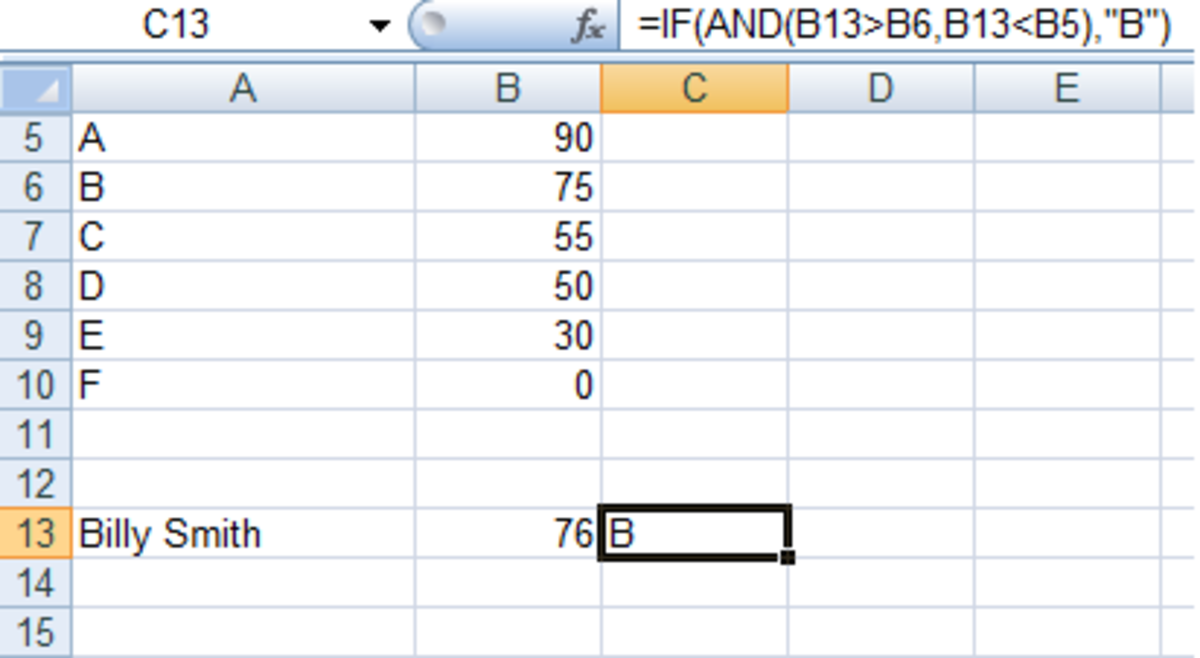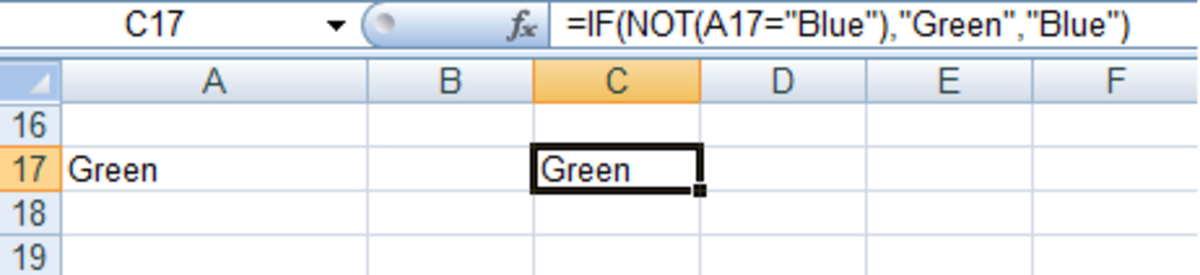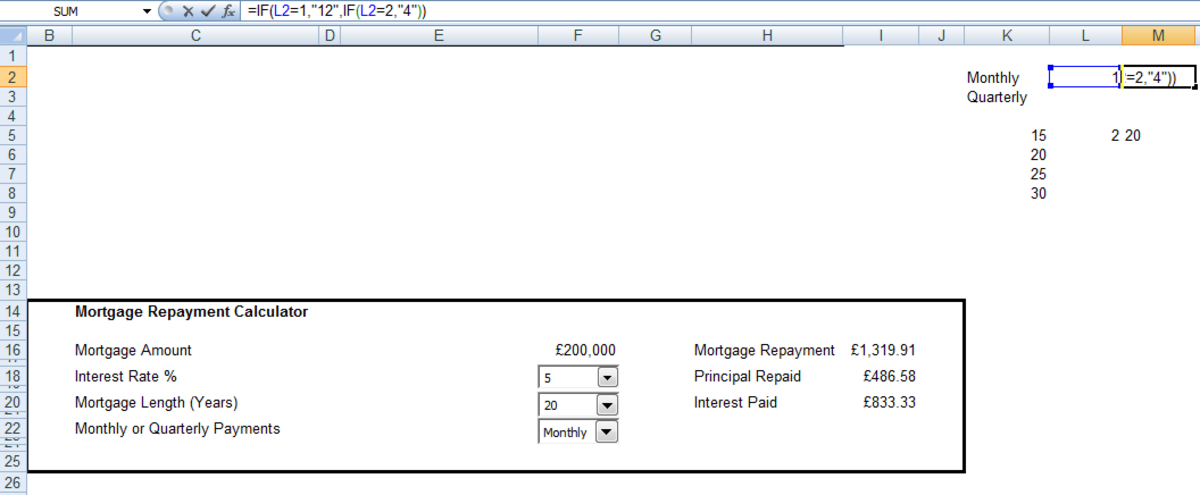# Using the IF and IFERROR Functions in Excel 2007 and 2010

Robbie mostly writes about Skyrim but also occasionally sheds light on the oddities of Microsoft applications such as Excel and Outlook.

Welcome to my latest article on Excel. Today I am going to look at the IF and IFERROR functions. The IF statement compares the cell you provide with what you tell Excel to look for and returns one result if it is true and another if it is false. The IFERROR function permits us to suppress expected errors in our spreadsheets.

The IF statement is made up of three elements:

• What it is Excel is looking for.
• What you want it to do if it is true.
• What to do if it is false.

If you don’t tell Excel what to do if it is false, Excel will just return FALSE as a result.

There are a number of situations where you may want to use an IF statement:

• When you are comparing the contents of your cell with something else, e.g., “Does the cell I am interested in contain the word Blue?”
• Calculating something based on the cell's contents, e.g., “Does Billy Smith’s exam results earn him a B grade?”
• Converting the contents of the cell to something else, e.g., “If B2=1, then I want C2 to display “Aries.”

We will work through examples of each as well as how to use IF with the AND, OR, and NOT functions.

• If we use IF and AND together, Excel will do one thing if all the conditions are met and the other if they are not all met.
• When using IF and OR, Excel will do one thing if one of the conditions is met and another if none are met.
• Using IF and NOT together, Excel will do one thing if the condition is not met and another if it is.

In addition, we will look at the IFERROR function, which allows us to suppress expected errors.

## Example of Using an IF Statement

To begin, we will look at a simple example of the IF statement. Here we are comparing the contents of the cell with the word blue.

=IF(A2="Blue", "Cell is Blue", "Cell is not Blue")Example of a simple IF statement in Excel 2007 and Excel 2010.http://robbiecwilson.hubpages.com/

## Using Multiple IF Statements

Suppose, rather than asking Excel to compare the cell with one thing, we want Excel to compare it against a number of things. We can use multiple IF statements in a formula. You can see an example of such a formula below:

=IF(L5=1,"15",IF(L5=2,"20",IF(L5=3,"25",IF(L5=4,"30"))))

Pay particular attention to the brackets in the above formula. Notice that all the open brackets are on the left and that all the closed brackets are on the right (the IF statements are separated by commas rather than closed brackets.) This nests the IF statements within each other.

Scroll to Continue

## Using IF With AND Function

Using the logical function AND with IF allows you to test the contents of your cell using more than one condition. We can use AND to determine if Billy has earned a B grade in his recent test. To gain a B, he needed to score between 75 and 90.

=IF(AND(B13>B6,B13<B5),"B")Using IF and AND in a formula in Excel 2007 and Excel 2010.http://robbiecwilson.hubpages.com/
• In the above formula, we look at the contents of cell B13.
• Using the AND function we check if it is greater than B6, which is the bottom boundary for the B grade, and less than B5, which is the top boundary for the B grade.
• And if it is between those two numbers, we assign that mark a B grade.

## Using IF With OR Function

Similar to AND, you use can use OR to test your cell against more than one condition. With OR, only one of the conditions has to be true for the IF statement to be true. For example, I would like to know if the content of cell A15 is cow or pig. If it is either of those, B15 will display mammal. If not, it will be blank.

=IF(OR(A15="Cow", A15="Pig"),"Mammal", "")

Excel looks at cell A15 and finds cow, it then looks at what it is supposed to be looking for which is cow or pig. As the condition is met, it will return “Mammal.” If it found chicken in cell A15, it would then return as chicken having not met the conditions of the OR function.

## Using IF With NOT Function

The final logical function we will look at is NOT. It looks at a single value and determines whether or not it is the same as the cell it is comparing it with. In my example, I have a cell that contains the word Green. Excel looks at that cell and compares it with the word Blue. If it is not blue it returns green and if it is blue it returns blue.Using IF with NOT in a formula in Excel 2007 and Excel 2010.http://robbiecwilson.hubpages.com/

## Converting the Contents of a Cell Using IF Statements

The third use of the IF statement in Excel is converting the contents of a cell into something else that we can use in a different way to its original contents. Often the result of a formula or the user's selection from a drop-down box is not in the format you need. You can use IF statements to convert it.

For example, I have a drop-down box that invites the user to select monthly or quarterly payments.

• The drop-down box places a 1 in cell L2 if the user selects monthly and 2 in L2 if the user selects quarterly.
• Excel needs the number to calculate the number of annual repayments for the user's mortgage and will be 4 if quarterly and 12 if monthly.

To achieve this, I use IF to convert 1 to 12 and 2 to 4 with the following formula:

=IF(L2=1,"12",IF(L2=2,"4"))Using IF to convert the contents of a cell in Excel 2007 and Excel 2010.http://robbiecwilson.hubpages.com/

Excel now uses the result which is stored in M2 to correctly calculate the formulas for the interest and principal paid each period.

## Using the IFERROR Function

The last function we will look at today is the IFERROR function. This is used if you want to change the way Excel behaves if it produces an error. Often, depending on what a user does, Excel may alert you to an error that is expected.

Suppose someone uses my mortgage calculator to look at a mortgage over 35 years. They then change their mind and choose a 20-year mortgage. All the cells between 21 and 35 years will now display an error (a #NUM error.) To suppress that error, we nest the original formula in IFERROR.

The original formula was:

=PPMT(\$R\$2/\$M\$2,A35,\$M\$2*\$M\$5,-\$F\$16)

We add IFERROR like so:

=IFERROR(PPMT(\$R\$2/\$M\$2,A35,\$M\$2*\$M\$5,-\$F\$16), " ")

What will now happen is that if there is an error in this cell, it will display a single space rather than an error.

You should ensure that your formula works perfectly before nesting using IFERROR. Otherwise, you will never know if it produces an error due to a typo or other mistake.

## Using IF and IFERROR Functions Together: In Summary

We looked at the IF and IFERROR functions and used them together with the logical functions AND, OR, and NOT. IF can be used:

• To compare a cell’s contents.
• To convert a cell to something else.
• And to perform a calculation on a cell based on what is in it.

Using the IF function we ask Excel to compare a cell with something we are interested in and do one thing if it is true and another if it is false. So if we have, for example, a cell containing the word parrot and we want Excel to look at that cell and return bird if the cell contains the word parrot and return not bird if it doesn’t then we can use IF to do this.

We also covered using multiple IF statements in one formula paying special attention to the brackets.

In combination with the other logical functions, we saw that:

• If we use IF and AND together, Excel will do one thing if all the conditions are met and the other if they are not all met.
• When using IF and OR. Excel will do one thing if one of the conditions is met and another if none are met.
• Using IF and NOT together, Excel will do one thing if the condition is not met and another if it is.

The last function we examined was the IFERROR function. This allows you to suppress an expected error or change the text that Excel displays when an error occurs.

Many thanks for reading, and I hope that you are now happily using the very versatile IF functions in your spreadsheets. Please feel free to leave any comments you may have below.

This article is accurate and true to the best of the author’s knowledge. Content is for informational or entertainment purposes only and does not substitute for personal counsel or professional advice in business, financial, legal, or technical matters.